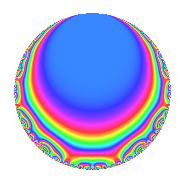# Properties

 Label 151.1.b.aLevel 151 Weight 1 Character orbit 151.b Self dual Yes Analytic conductor 0.075 Analytic rank 0 Dimension 3 Projective image $$D_{7}$$ CM disc. -151 Inner twists 2

# Related objects

## Newspace parameters

 Level: $$N$$ = $$151$$ Weight: $$k$$ = $$1$$ Character orbit: $$[\chi]$$ = 151.b (of order $$2$$ and degree $$1$$)

## Newform invariants

 Self dual: Yes Analytic conductor: $$0.0753588169076$$ Analytic rank: $$0$$ Dimension: $$3$$ Coefficient field: $$\Q(\zeta_{14})^+$$ Coefficient ring: $$\Z[a_1, a_2]$$ Coefficient ring index: $$1$$ Projective image $$D_{7}$$ Projective field Galois closure of 7.1.3442951.1 Artin image size $$14$$ Artin image $D_7$ Artin field Galois closure of 7.1.3442951.1

## $q$-expansion

Coefficients of the $$q$$-expansion are expressed in terms of a basis $$1,\beta_1,\beta_2$$ for the coefficient ring described below. We also show the integral $$q$$-expansion of the trace form.

 $$f(q)$$ $$=$$ $$q -\beta_{1} q^{2} + ( 1 + \beta_{2} ) q^{4} + ( -1 + \beta_{1} - \beta_{2} ) q^{5} + ( -1 - \beta_{2} ) q^{8} + q^{9} +O(q^{10})$$ $$q -\beta_{1} q^{2} + ( 1 + \beta_{2} ) q^{4} + ( -1 + \beta_{1} - \beta_{2} ) q^{5} + ( -1 - \beta_{2} ) q^{8} + q^{9} + ( -1 + \beta_{1} ) q^{10} + \beta_{2} q^{11} + \beta_{1} q^{16} + \beta_{2} q^{17} -\beta_{1} q^{18} -\beta_{1} q^{19} - q^{20} + ( -1 - \beta_{2} ) q^{22} + ( 1 - \beta_{1} ) q^{25} -\beta_{1} q^{29} + ( -1 + \beta_{1} - \beta_{2} ) q^{31} - q^{32} + ( -1 - \beta_{2} ) q^{34} + ( 1 + \beta_{2} ) q^{36} + \beta_{2} q^{37} + ( 2 + \beta_{2} ) q^{38} + q^{40} + ( -1 + \beta_{1} - \beta_{2} ) q^{43} + ( 1 + \beta_{1} ) q^{44} + ( -1 + \beta_{1} - \beta_{2} ) q^{45} -\beta_{1} q^{47} + q^{49} + ( 2 - \beta_{1} + \beta_{2} ) q^{50} + ( -\beta_{1} + \beta_{2} ) q^{55} + ( 2 + \beta_{2} ) q^{58} + ( -1 + \beta_{1} - \beta_{2} ) q^{59} + ( -1 + \beta_{1} ) q^{62} + ( 1 + \beta_{1} ) q^{68} + ( -1 - \beta_{2} ) q^{72} + ( -1 - \beta_{2} ) q^{74} + ( -1 - \beta_{1} - \beta_{2} ) q^{76} + ( 1 - \beta_{1} ) q^{80} + q^{81} + ( -\beta_{1} + \beta_{2} ) q^{85} + ( -1 + \beta_{1} ) q^{86} + ( -1 - \beta_{1} ) q^{88} + ( -1 + \beta_{1} ) q^{90} + ( 2 + \beta_{2} ) q^{94} + ( -1 + \beta_{1} ) q^{95} -\beta_{1} q^{97} -\beta_{1} q^{98} + \beta_{2} q^{99} +O(q^{100})$$ $$\operatorname{Tr}(f)(q)$$ $$=$$ $$3q - q^{2} + 2q^{4} - q^{5} - 2q^{8} + 3q^{9} + O(q^{10})$$ $$3q - q^{2} + 2q^{4} - q^{5} - 2q^{8} + 3q^{9} - 2q^{10} - q^{11} + q^{16} - q^{17} - q^{18} - q^{19} - 3q^{20} - 2q^{22} + 2q^{25} - q^{29} - q^{31} - 3q^{32} - 2q^{34} + 2q^{36} - q^{37} + 5q^{38} + 3q^{40} - q^{43} + 4q^{44} - q^{45} - q^{47} + 3q^{49} + 4q^{50} - 2q^{55} + 5q^{58} - q^{59} - 2q^{62} + 4q^{68} - 2q^{72} - 2q^{74} - 3q^{76} + 2q^{80} + 3q^{81} - 2q^{85} - 2q^{86} - 4q^{88} - 2q^{90} + 5q^{94} - 2q^{95} - q^{97} - q^{98} - q^{99} + O(q^{100})$$

Basis of coefficient ring in terms of $$\nu = \zeta_{14} + \zeta_{14}^{-1}$$:

 $$\beta_{0}$$ $$=$$ $$1$$ $$\beta_{1}$$ $$=$$ $$\nu$$ $$\beta_{2}$$ $$=$$ $$\nu^{2} - 2$$
 $$1$$ $$=$$ $$\beta_0$$ $$\nu$$ $$=$$ $$\beta_{1}$$ $$\nu^{2}$$ $$=$$ $$\beta_{2} + 2$$

## Character Values

We give the values of $$\chi$$ on generators for $$\left(\mathbb{Z}/151\mathbb{Z}\right)^\times$$.

 $$n$$ $$6$$ $$\chi(n)$$ $$-1$$

## Embeddings

For each embedding $$\iota_m$$ of the coefficient field, the values $$\iota_m(a_n)$$ are shown below.

For more information on an embedded modular form you can click on its label.

Label $$\iota_m(\nu)$$ $$a_{2}$$ $$a_{3}$$ $$a_{4}$$ $$a_{5}$$ $$a_{6}$$ $$a_{7}$$ $$a_{8}$$ $$a_{9}$$ $$a_{10}$$
150.1
 1.80194 0.445042 −1.24698
−1.80194 0 2.24698 −0.445042 0 0 −2.24698 1.00000 0.801938
150.2 −0.445042 0 −0.801938 1.24698 0 0 0.801938 1.00000 −0.554958
150.3 1.24698 0 0.554958 −1.80194 0 0 −0.554958 1.00000 −2.24698
 $$n$$: e.g. 2-40 or 990-1000 Significant digits: Format: Complex embeddings Normalized embeddings Satake parameters Satake angles

## Inner twists

Char. orbit Parity Mult. Self Twist Proved
1.a Even 1 trivial yes
151.b Odd 1 CM by $$\Q(\sqrt{-151})$$ yes

## Hecke kernels

There are no other newforms in $$S_{1}^{\mathrm{new}}(151, [\chi])$$.